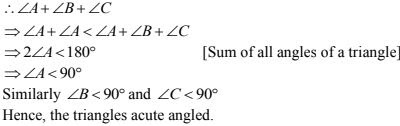# Chapter 9 Triangles and its Angles RD Sharma Solutions Exercise 9.1 Class 9 Maths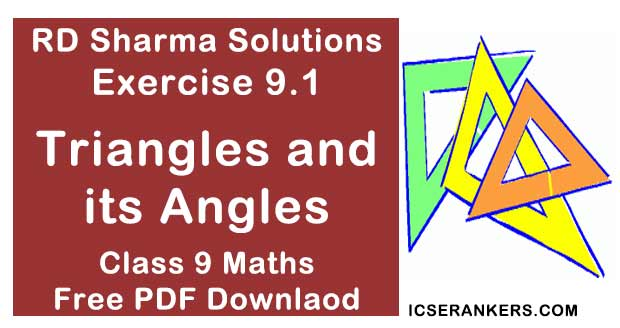Chapter Name RD Sharma Chapter 9 Triangles and its Angles Exercise 9.1 Book Name RD Sharma Mathematics for Class 10 Other Exercises No Other Exercises Related Study NCERT Solutions for Class 10 Maths

### Exercise 9.1 Solutions

1. In a ΔABC, if ∠A = 55°, ∠B = 40° , find ∠C.

Solution

Given ∠A = 55° , ∠B = 40 then ∠C = ?
We know that
In  a ΔABC sum of all angles of triangle is 180°
i.e., ∠A + ∠B + ∠C = 180°
⇒ 55° + 40° + ∠C = 180°
⇒ 95° + ∠C = 180°
⇒ ∠C = 180° - 95°
⇒ ∠C = 85°

2. If the angles of a triangles are in the ratio 1 : 2 : 3, determine three angles.

Solution

Given that the angles of a triangle are in the ratio 1 : 2 : 3
Let the angles be a, 2a, 3a
∴ We know that
Sum of all angles of triangles is 80°
a + 2a + 3a = 180°
⇒ 6a = 180°
⇒ a = 180°/6
⇒ a = 30°
Since a = 30°
2a = 2(30°) = 60°
3a = 3(30°) = 90°
∴ angles are a = 30°, 2a = 60°, 3a = 90°
∴ Hence angles are 30°, 60° and 90°

3. The angles of a triangle are (x - 40)° , (x - 20)° and (x/2 - 10)° . Find the value of x.

Solution

Given that
The angles of a triangle are
(x - 40)° , (x - 20)°  and (x/2 - 10)°
We know that
Sum of all angles of triangle is 180°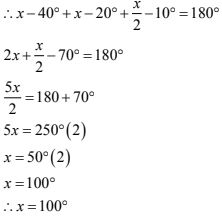4. The angles of a triangle are arranged in ascending order of magnitude. If the difference between two consecutive angles is 10° , find the three angles.

Solution

Given that,
The difference between two consecutive angles is  10° .
Let x, x + 10, x + 20 be the consecutive angles differ by  10°
W.K.T sum of all angles of triangle is 180° .
x + x + 10 + x + 20 = 180°
3x + 30 = 180°
⇒ 3x = 180 - 30°
⇒ 3x = 150°
⇒ x = 50°
x = 50°
The required angles are
x, x + 10 and x + 20
x = 50°
⇒ x + 10 = 50 + 10 = 60
⇒ x + 20 = 50 + 10 + 10 = 70
The difference between two consecutive angles is 10° then three angles are 50°, 60° and 70°.

5. Two angles of a triangle are equal and the third angle is greater than each of those angles by 30°. Determine all the angles of the triangle.

Solution

Given that,
Two angles are equal and the third angle is greater than each of those angles by  30° .
Let x, x, x + 30 be the angles of a triangle
We know that
Sum of all angles of a triangle is 180°
x + x + x + 30 = 180°
3x + 30 = 180°
⇒ 3x = 180° - 30°
⇒ 3x = 150°
⇒ x = 150°/3
⇒ x = 50°
∴ The angles are x, x, x + 30
x = 50°
⇒ x + 30 = 80°
∴ The required angles are 50°, 50°, 80°.

6. If one angle of a triangle is equal to the sum of the other two, show that the triangle is a right triangle.
Solution
If one angle of a triangle is equal to the sum of other two
i.e., ∠B = ∠A + ∠C
Now, in ΔABC
(Sum of all angles of triangle 180°)
∠A + ∠B + ∠C = 180°
⇒ ∠B + ∠B = 180° [∵ ∠B = ∠A + ∠C]
⇒ 2∠B = 180°
⇒ ∠B = 180°/2
⇒ ∠B = 90°
∴ ABC is a right angled a triangle.

7.  ABC is a triangle in which ∠A -72° , the internal bisectors of angles B and C meet in O.
Find the magnitude of ∠ROC.
Solution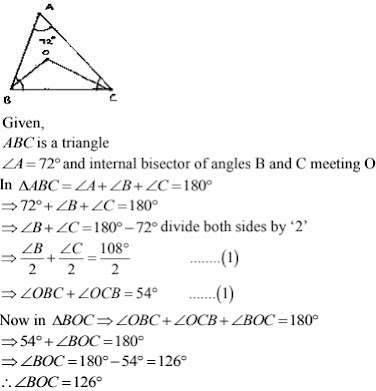8. The bisectors of base angles of a triangle cannot enclose a right angle in any case.
Solution
In a  ΔABC
Sum of all angles of triangles is 180°
i.e.,  ∠A + ∠B + ∠C = 180° divide both sides by '2' .9. If the bisectors of the base angles of a triangle enclose an angle of 135°, prove that the triangle is a right triangle.
Solution
Given the bisectors the base angles of an triangle enclose an angle of  135°
i.e., ∠BOC = 135°
But, W.K.T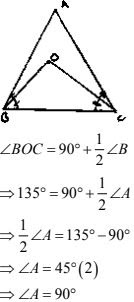∴ ΔABC is right angled triangle right angled at A.

10. In a ΔABC, ∠ABC = ∠ACB and the bisectors of ∠ABC and ∠ACB intersect at O such that ∠BOC = 120. Show that ∠A = ∠B = ∠C = 60°.
Solution
Given,
In ΔABC
∠ABC = ∠ACB
Divide both sides by '2'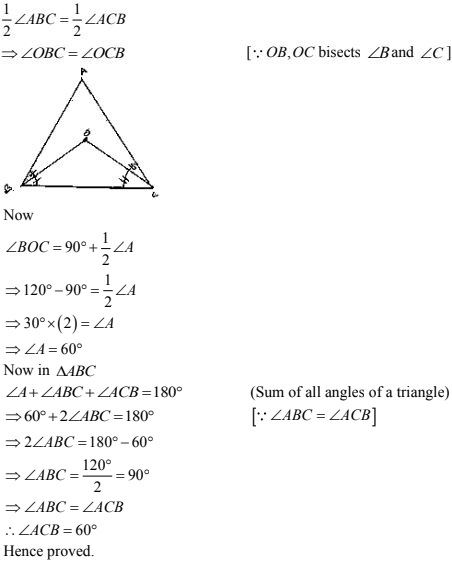11. Can a triangle have :
(i) Two right angles ?
(ii)  Two obtuse angles ?
(iii) Two acute angles ?
(iv) All angles more than 60° ?
(v) All angles less than 60° ?
(vi) All angles equal to 60° ?
Solution
(i) No
Two right angles would up to 180°. So, the third angle becomes zero. This is not possible, so a triangle cannot have two right angles.
[Since sum of angles in a triangle is 180°]

(ii) No
A triangle can’t have 2 obtuse angles. Obtuse angle means more than 90°. So, that the sum of the two sides will exceed 180° which is not possible. As the sum of all three angles of a triangle is 180°.

(iii) Yes
A triangle can have 2 acute angle. Acute angle means less the 90° angle.

(iv) No
Having angles-more than 60° make that sum more than 180° which is not possible.
[∵ The sum of all the internal angles of a triangle is 180°]

(v) No
Having all angles less than 60° will make that sum less than 180° which is not possible.
[∵ The sum of all the internal angles of a triangle is 180°.]

12. If each angle of a triangle is less than the sum of the other two, show that the triangle is acute angled.
Solution
Given each angle of a triangle less than the sum of the other two